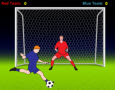# Soccer Math GamesKids all over the world love playing soccer. Why not incorporate this fun sport to learn or practice new math skills? These online games are educational and a lot of fun in. Drills alone are boring, although students need a lot of practice to become more proficient in math.

These games can help students stay interested in learning new math skills.

Practice your math skills by playing these fun soccer math games!

The following soccer math games are suitable for elementary students:

Addition and Subtraction Soccer Game (New)
Elementary students will practice writing small numbers as sums and differences of other numbers.

In this game students will add two-digit whole numbers.

Identify and apply the properties of addition by playing an interactive soccer game.

Subtracting Two-Digit Whole Numbers
Students will subtract two-digit whole numbers. The problems must be answered correctly in order to be given a chance to kick the ball.

In this fun game, kids will practice adding decimals and earn tons of points by scoring goals.

Subtracting Decimals Game
Solve each problem correctly to kick the ball.

Simplifying Fractions Game
Reduce each fraction to the simplest form.

Rounding Decimals Game
Round each decimal to the nearest whole number.

Multiplying Fractions Game
Multiply fractions and write the answer in simplest form.

Division With Remainder(New)
Practice division with remainder problems (3 and 4-digit dividend; one-digit divisor.)

Dividing Fractions Game
Divide fractions and write the answer in simplest form.

One-Step Equations Game
Solve one-step equations with addition and subtraction.

Return from the Soccer Math Games page to the Math Play homepage.